# NCERT Solutions for Class 10 Maths Exercise 3.3## myCBSEguide App

CBSE, NCERT, JEE Main, NEET-UG, NDA, Exam Papers, Question Bank, NCERT Solutions, Exemplars, Revision Notes, Free Videos, MCQ Tests & more.

NCERT solutions for Maths Pair of Linear Equations in Two Variables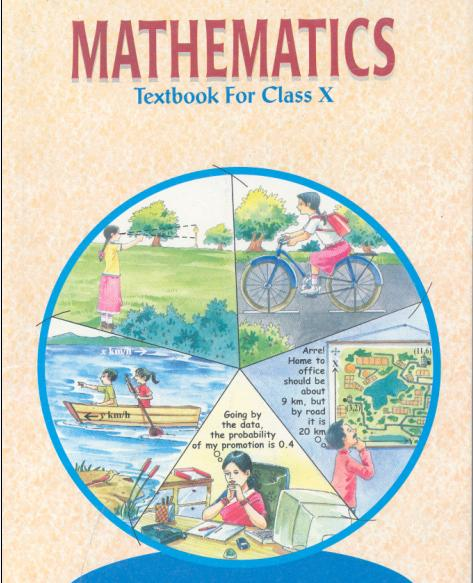## NCERT Solutions for Class 10 Maths Pair of Linear Equations in Two Variables

1. Solve the following pair of linear equations by the substitution method.

(i) x + y = 14

x y = 4

(ii) s t = 3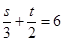(iii) 3x – y = 3

9x − 3y = 9

(iv)0.2x + 0.3y = 1.3

0.4x + 0.5y = 2.3

(v)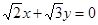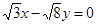(vi)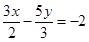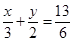Ans. (i) x + y = 14 …(1)

x y = 4 … (2)

x = 4 + y from equation (2)

Putting this in equation (1), we get

4 + y + y = 14

⇒ 2y = 10⇒ y = 5

NCERT Solutions for Class 10 Maths Exercise 3.3

Putting value of y in equation (1), we get

x + 5 = 14

x = 14 – 5 = 9

Therefore, x = 9 and y = 5

(ii) s t = 3 … (1)(2)

Using equation (1), we can say that s = 3 + t

Putting this in equation (2), we get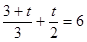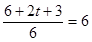⇒ 5t + 6 = 36

⇒ 5t = 30⇒ t = 6

Putting value of t in equation (1), we get

s – 6 = 3⇒ s = 3 + 6 = 9

Therefore, t = 6 and s = 9

(iii) 3x y = 3 … (1)

9x − 3y = 9 … (2)

Comparing equation 3x y = 3 with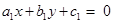and equation 9x − 3y = 9 with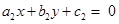,

We get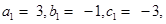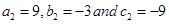Here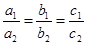Therefore, we have infinite many solutions for x and y

(iv) 0.2x + 0.3y = 1.3 … (1)

0.4x + 0.5y = 2.3 … (2)

Using equation (1), we can say that

0.2x = 1.3 − 0.3y

x =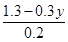Putting this in equation (2), we get

0.4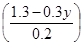+ 0.5y = 2.3

⇒ 2.6 − 0.6y + 0.5y = 2.3

⇒ −0.1y = −0.3 ⇒ y = 3

Putting value of y in (1), we get

0.2x + 0.3 (3) = 1.3

⇒ 0.2x + 0.9 = 1.3

⇒ 0.2x = 0.4 ⇒ x = 2

Therefore, x = 2 and y = 3

(v)……….(1)……….(2)

Using equation (1), we can say that

x =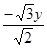NCERT Solutions for Class 10 Maths Exercise 3.3

Putting this in equation (2), we get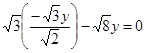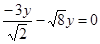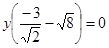y = 0

Putting value of y in (1), we get x = 0

Therefore, x = 0 and y = 0

(vi)… (1)… (2)

Using equation (2), we can say that

x =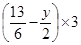x =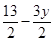Putting this in equation (1), we get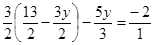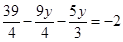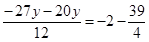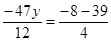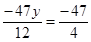y = 3

Putting value of y in equation (2), we get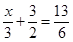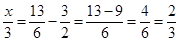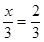⇒ x = 2

Therefore, x = 2 and y = 3

NCERT Solutions for Class 10 Maths Exercise 3.3

###### 2. Solve 2x + 3y = 11 and 2x − 4y = −24 and hence find the value of ‘m’ for which

y = mx + 3.

Ans. 2x + 3y = 11 … (1)

2x − 4y = −24 … (2)

Using equation (2), we can say that

2x = −24 + 4y

x = −12 + 2y

Putting this in equation (1), we get

2 (−12 + 2y) + 3y = 11

⇒ −24 + 4y + 3y = 11

⇒ 7y = 35 ⇒ y = 5

Putting value of y in equation (1), we get

2x + 3 (5) = 11

⇒ 2x + 15 = 11

⇒ 2x = 11 – 15 = −4⇒ x = −2

Therefore, x = −2 and y = 5

Putting values of x and y in y = mx + 3, we get

5 = m (−2) + 3

⇒ 5 = −2m + 3

⇒ −2m = 2 ⇒ m = −1

NCERT Solutions for Class 10 Maths Exercise 3.3

###### 3. Form a pair of linear equations for the following problems and find their solution by substitution method.

(i) The difference between two numbers is 26 and one number is three times the other. Find them.

(ii) The larger of two supplementary angles exceeds the smaller by 18 degrees. Find them.

(iii)The coach of a cricket team buys 7 bats and 6 balls for Rs 3800. Later, she buys 3 bats and 5 balls for Rs 1750. Find the cost of each bat and each ball.

(iv) The taxi charges in a city consist of a fixed charge together with the charge for the distance covered. For a distance of 10 km, the charge paid is Rs 105 and for a journey of 15 km, the charge paid is Rs 155. What are the fixed charges and the charge per km? How much does a person have to pay for travelling a distance of 25 km?

(v) A fraction becomes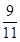, if 2 is added to both the numerator and the denominator. If, 3 is added to both the numerator and denominator it becomes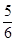. Find the fraction.

(vi) Five years hence, the age of Jacob will be three times that of his son. Five years ago, Jacob’s age was seven times that of his son. What are their present ages?

Ans. (i) Let first number be x and second number be y.

According to given conditions, we have

x y = 26 (assuming x > y) … (1)

x = 3y(x > y)… (2)

Putting equation (2) in (1), we get

3y y = 26

⇒ 2y = 26

y = 13

Putting value of y in equation (2), we get

x = 3y =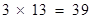Therefore, two numbers are 13 and 39.

(ii) Let smaller angle =x and let larger angle =y

According to given conditions, we have

y = x + 18 … (1)

Also,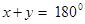(Sum of supplementary angles) … (2)

Putting (1) in equation (2), we get

x + x + 18 = 180

⇒ 2x = 180 – 18 = 162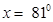Putting value of x in equation (1), we get

y = x + 18 = 81 + 18 =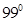Therefore, two angles are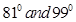.

NCERT Solutions for Class 10 Maths Exercise 3.3

(iii) Let cost of each bat = Rs x and let cost of each ball = Rs y

According to given conditions, we have

7x + 6y = 3800 … (1)

And,3x + 5y = 1750 … (2)

Using equation (1), we can say that

7x = 3800 − 6y x =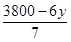Putting this in equation (2), we get

3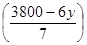+ 5y = 1750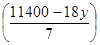+ 5y = 1750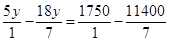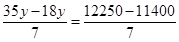⇒ 17y = 850 ⇒ y = 50

Putting value of y in (2), we get

3x + 250 = 1750

⇒ 3x = 1500 ⇒ x = 500

Therefore, cost of each bat = Rs 500 and cost of each ball = Rs 50

(iv) Let fixed charge = Rs x and let charge for every km = Rs y

According to given conditions, we have

x + 10y = 105… (1)

x + 15y = 155… (2)

Using equation (1), we can say that

x = 105 − 10y

Putting this in equation (2), we get

105 − 10y + 15y = 155

⇒ 5y = 50 ⇒ y = 10

Putting value of y in equation (1), we get

x + 10 (10) = 105

x = 105 – 100 = 5

Therefore, fixed charge = Rs 5 and charge per km = Rs 10

To travel distance of 25 Km, person will have to pay = Rs (x + 25y)

= Rs (5 + 25 × 10)

= Rs (5 + 250) = Rs 255

NCERT Solutions for Class 10 Maths Exercise 3.3

(v) Let numerator = x and let denominator = y

According to given conditions, we have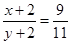… (1)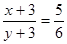… (2)

Using equation (1), we can say that

11 (x + 2) = 9y + 18

⇒ 11x + 22 = 9y + 18

⇒ 11x = 9y – 4

x =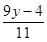Putting value of x in equation (2), we get

6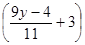= 5 (y + 3)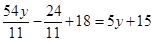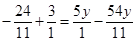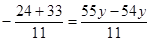y = 9

Putting value of y in (1), we get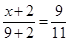x + 2 = 9 ⇒ x = 7

Therefore, fraction =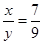(vi) Let present age of Jacob = x years

Let present age of Jacob’s son = y years

According to given conditions, we have

(x + 5) = 3 (y + 5) … (1)

And, (x − 5) = 7 (y − 5) … (2)

From equation (1), we can say that

x + 5 = 3y + 15

x = 10 + 3y

NCERT Solutions for Class 10 Maths Exercise 3.3

Putting value of x in equation (2) we get

10 + 3y – 5 = 7y − 35

⇒ −4y = −40

y = 10 years

Putting value of y in equation (1), we get

x + 5 = 3 (10 + 5) =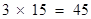x = 45 – 5 = 40 years

Therefore, present age of Jacob = 40 years and, present age of Jacob’s son = 10 years

## NCERT Solutions for Class 10 Maths Exercise 3.3

NCERT Solutions Class 10 Maths PDF (Download) Free from myCBSEguide app and myCBSEguide website. Ncert solution class 10 Maths includes text book solutions from Mathematics Book. NCERT Solutions for CBSE Class 10 Maths have total 15 chapters. 10 Maths NCERT Solutions in PDF for free Download on our website. Ncert Maths class 10 solutions PDF and Maths ncert class 10 PDF solutions with latest modifications and as per the latest CBSE syllabus are only available in myCBSEguide.

## CBSE app for Class 10

To download NCERT Solutions for Class 10 Maths, Computer Science, Home Science,Hindi ,English, Social Science do check myCBSEguide app or website. myCBSEguide provides sample papers with solution, test papers for chapter-wise practice, NCERT solutions, NCERT Exemplar solutions, quick revision notes for ready reference, CBSE guess papers and CBSE important question papers. Sample Paper all are made available through the best app for CBSE students and myCBSEguide website.### 30 thoughts on “NCERT Solutions for Class 10 Maths Exercise 3.3”

1. Good

2. It’s very nice for we students of class 10

3. Helpful to all tenth students

6. Very nice guru ji

7. Sir thanks for help me

8. Nice

9. Very helpful for me thank u ??

10. Thank you so much for giving easy method to understand by which a student can understand easily and clearly. I am satisfying with this answer.

11. Best solution ever to be explained online

12. It’s nice and helpful to each and every student of 10 with correct answers thanks a lot

13. Thanks to help

14. Sir . I always owe for u,, I m completely satisfied with the above solution …. again tlhanks alot … thnks very much

15. Nice job by CBSE guide

16. Nice

17. Thanx it was helpful for me.

18. A wonderful work

19. Thank you for helping me

20. Good I loved it .???so useful

21. I am love ??it

It is very useful app

22. I am love ??it

It is very useful app

23. Thanks !
It I very useful for me so thanks

24. Very useful to me. Thanks u so much.??

25. thank you for helping me

26. thank you for helping me as well as class 10

27. good

28. Thankyou very much

29. It’s really amazing. I Love it.